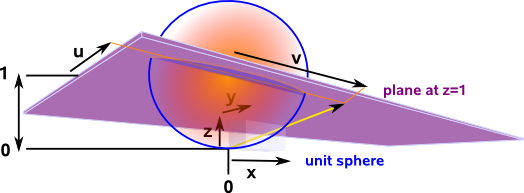# Maths - Stereographic Model

In the projective domain the length of the vector is less important because the projection onto the euclidean domain depends on the direction of the vector and not its length. There are different ways to deal with this redundant information about the length of the vector in the projective plane. We can call these models:

• hemisphere - normalise it to unit sphere centred at origin.
• stereographic - here the sphere is centered on the plane (0,0,1) and we project from the origin which is a point on the surface of the sphere.

here we look at the properties of the stereographic model.

In order to illustrate the properties of this model we will use a two dimensional plane mapped onto three dimensional projective space.Here (u,v) represents the coordinates on the plane and (x,y,z) represents the coordinates in projective space (normalised to a unit sphere at z=1).

So to convert from euclidean coordinates to projective (stereographic model) coordinates we have:

 x y z
= 2/(1 + u² + v²)
 u v 1

and to convert from projective (hemisphere model) coordinates to euclidean coordinates we have:

 u v
=
 x/z y/z

Both these mappings are derived for this two dimensional case on this page.

On the plot below we have put a rectangular grid on the euclidean plane, there is an error with the uv texture coordinates in the diagram below so when this is plotted onto projective space the vertical and horizontal lines no longer meet each other at 90° so the space does not appear to be conformal although is should be? I need to do some more work on this.## Infinity

Let us take the 2D (3D in projective space) case.

First horizontal lines, in this case u=∞ which gives,

 x y z
= 1/(1+u²+v²)
 u v 1
=
 u/(1+u²+v²) v/(1+u²+v²) 1/(1+u²+v²)
=
 1/(1/u+u+v²/u) v/u*(1/u+u+v²/u) 1/u*(1/u+u+v²/u)

so substituting u=∞ gives,

=
 1/(0+∞+0) v/∞(0+∞+0) 1/∞(0+∞+0)
=
 0 0 0
so for horizontal lines they meet at:
 0 0 0

For vertical lines, in this case v=∞ which gives,

 x y z
= 1/√(1+u²+v²)
 u v 1
=
 u/(1+u²+v²) v/(1+u²+v²) 1/(1+u²+v²)
=
 u/v*(1/v+u²/v + 1) 1/(1/v+u²/v + 1) 1/v*(1/v+u²/v + 1)

so substituting v=∞ gives,

=
 1/∞√(0+∞+0) v/√(0+∞+0) 1/∞√(0+∞+0)
=
 0 0 0
so for vertical lines they meet at:
 0 0 0

So in the stereographic projection infinity is the same for all directions of line (unlike hemisphere model).

## Special Cases

• For 1D projection in 2D space see page here.
• For 2D projection in 3D space see page here.Roger Penrose - The Road to Reality: Partly a 'popular science' book as it tries to minimise the number of equations (Not that I'm complaining much his book 'Spinors and space-time' went over my head in the first few pages) it still has lots of interesting results that its difficult to find elsewhere.Spinors and Space-Time: Volume 1, Two-Spinor Calculus and Relativistic Fields (Cambridge Monographs on Mathematical Physics) by Roger Penrose and Wolfgang Rindler - This book is about the mathematics of special relativity, it very quickly goes over my head by I hope I will understand it one day.